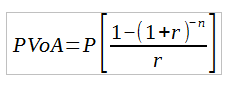# Present Value Annuity Assignment Help

## Basics of the present value annuity

An annuity is a series of equal dollar payments that are made for a specified number of years. In general “ordinary annuities” with a payment occurring at the end of each period is what people mean when referring to an annuity. An “annuity due” is one in which the payments occur at the beginning of each period. The present value of annuity equation determines the value of a series of future periodic payments at the current time and relies on the concept of time value of money. The concept of time value is that one dollar today is worth more than it will be at a future date. The present value of annuity formula is shown here:P is periodic payment, r is the rate per period and n is the number of periods. It is important to make sure that the rate is consistent with the other variables in the formula. If payments are monthly then the rate needs to be per month. For example if an annuity has a 12% annual rate and payments are made every month, then a monthly rate of 1% would need to be used. If payments were made annually then the 12 % rate would be used in the equation.

## Get help with your present value annuity assignment

The present value of annuity equation can look intimidating and the concepts behind it may be difficult to grasp without assistance. Our finance homework service can provide the present value assignment help you need. We specialize in assisting students with any type of finance homework assignment. In addition we offer finance test preparation help and individual tutoring services if you require them. Anybody can help you get the solution to a problem but we do much more. When you come to us for profitability index assignment help or present value annuity assignment help we work with you to ensure you understand how solutions are arrived at so you can confidently work with the equations and concepts on your own. We show the detailed calculations involved and provide explanations for each step to be sure you completely understand every aspect of the assigned topic.